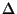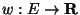Next: Approximate algorithms and approximation Up: Introduction Previous: NPO problems: definitions and   Index

### Terminology for graph problems

We will use V as the set of vertices, and E as the set of edges.is the max degree of the graph.

Although we focus on unweighted problems for reasons of succinctness, most problems have natural weighted variants. For instance, the solutions to the greater part of graph problems are in the form of a subset of the input, either edge or vertex subsets. In this case, instances of the problem are augmented with a weight function, on the edges (respectively,), and the objective function is modified to involve the sum of the weights of the edges (vertices) in the solution instead of their count. We will simply refer to this as the edge-weighted (vertex-weighted) case.

Similar situations hold for set problems, with weights on the sets or on the elements, and for satisfiability problems, with weights on the clauses or on the variables.

Viggo Kann
2000-03-20